Special Relativity

Take a moment away from reading this, and flip the nearest light switch. Unless you are superhuman, you did not detect the delay between the switch closing and the bulb lighting up. It turns out that if you owned a research-grade atomic clock, you would be able to measure a slight delay of tens of nanoseconds as the light travels from the bulb to your retina.

Brilliant challenge: build an atomic clock and measure this.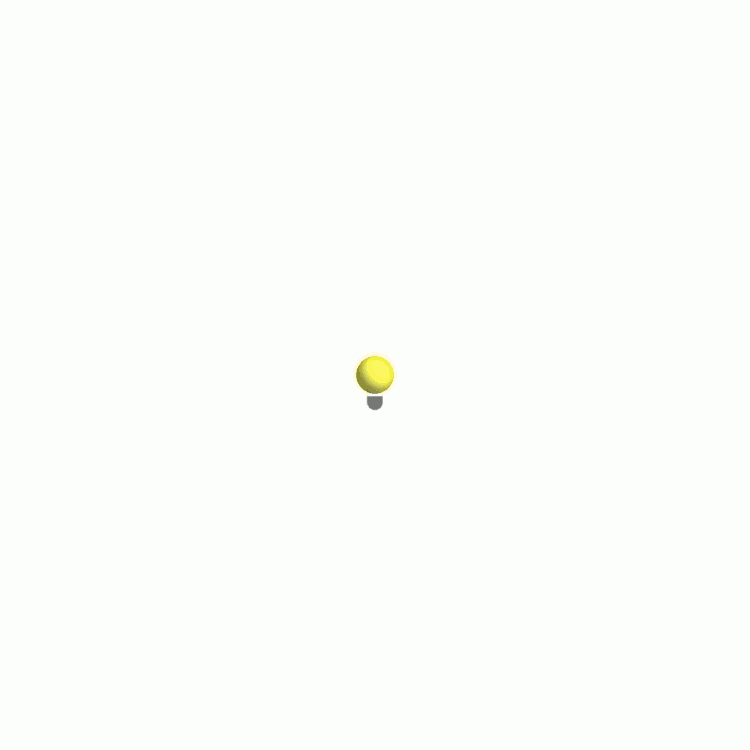Light travels at a finite speed, $c,$ which is large compared to speeds we typically encounter. The first person to suggest that light has a finite speed was Danish astronomer Ole Rømer while measuring the orbital period of Io—one of Jupiter's moons. He discovered systematic differences in Io's period depending on the time of year he made his observations, which he (quite radically) presented as evidence that light propagation is not instantaneous.

In this quiz, we will use our new tool, the spacetime diagram, to understand how the first estimate of $c$ was made shortly before the year 1700. Rømer's leap of intuition sowed the relativistic fruits that Einstein eventually reaped.

Propagation of Light

Rømer reasoned that if light propagates at a finite speed, then light from Io and Jupiter may take a significant time to reach Earth. However, no one had the faintest clue about the speed-of-light's magnitude, so Rømer couldn't say with any certainty whether the time delay between Jupiter and Earth was even measurable.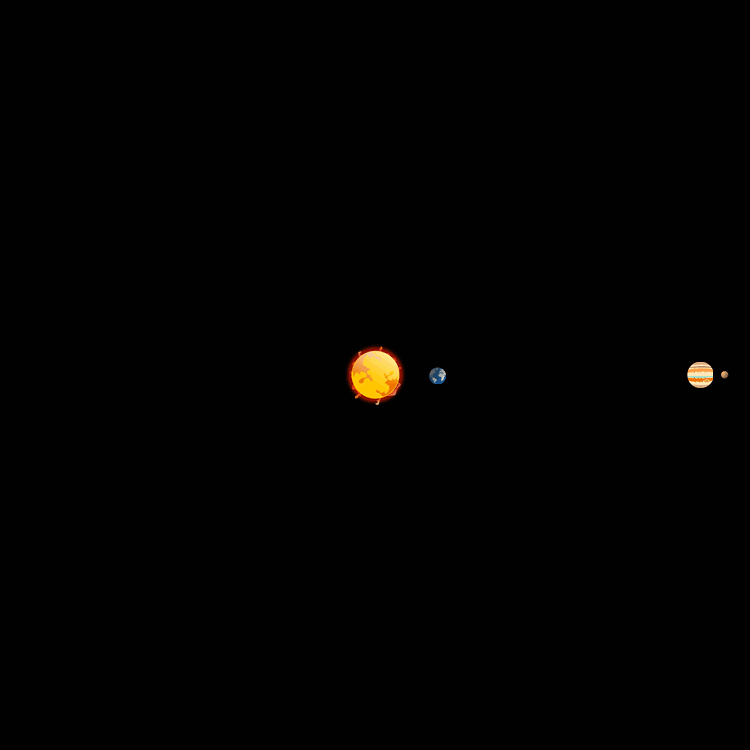Earth and Jupiter orbiting the Sun, while Io orbits Jupiter. (Note: this animation is not drawn to scale)

Earth and Jupiter revolve around the Sun. Their relative motion is described by some complicated function, and is not something we'd want to represent on a spacetime diagram.

However, Io orbits Jupiter with a period of about $T_\text{Io}=\SI{42}{\hour},$ which is much shorter than either Jupiter's or Earth's orbital period. Thus, during one revolution of Io, the distance between Earth and Jupiter does not change by much, relatively speaking. This fact can simplify spacetime diagrams representing light propagating to Earth during one revolution of Io.

Propagation of Light

To start, let's imagine both Jupiter and Earth are stationary and ignore their relative motion. Each time Io completes an orbit around Jupiter, a light beam propagates from Jupiter toward Earth (representing the light reflected from Io's surface that Rømer would see with his telescope).

The time between when these light beams arrive on Earth, $T_\text{obs},$ is the length of Io's period, as observed by Rømer.

How does $T_\textrm{obs}$ compare to Io's actual orbital period $T_\textrm{Io}?$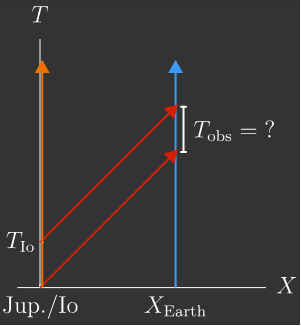Note: The red worldlines are light propagating with speed $c$ (which is the symbol most often used to represent light's speed) from Jupiter to Earth. Using the result from the previous quiz, the slope of these worldlines is $\text{slope}=\frac{\Delta T}{\Delta X}=\frac{1}{c}.$

Propagation of Light

Rømer knew that Earth and Jupiter do not usually share a rest frame. Earth is almost always moving away from Jupiter or moving toward it.

Suppose Jupiter is at rest in a reference frame, but now Earth is moving directly toward it. The spacetime diagram over one period of Io would now look slightly different.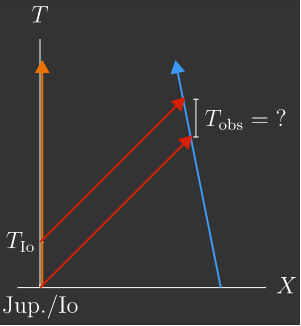Is the period of Io observed by someone moving directly toward Jupiter longer or shorter than the actual period $T_\textrm{Io}?$

Propagation of Light

In this course, worldlines on spacetime diagrams will guide us through the mind-bending universe of relativistic phenomena, so we'll try to prepare for this by using the spacetime diagram to work out $T_\text{obs}$ in terms of the actual period $T_\text{Io}.$

In relativity, we would say that Rømer's measurement of $T_\textrm{obs}$ is the time between two events. In this case, the events, which correspond to points on the spacetime diagram, are as follows:

• Event 1: Rømer starts his measurement of Io's period, logging where Io is in its orbit.
• Event 2: Rømer sees Io return to its initial position, so he stops his measurement.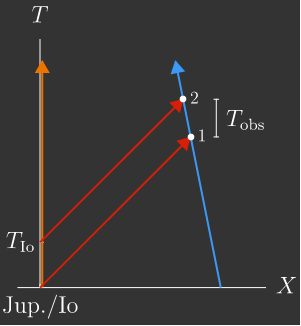The period that Rømer observes $T_\textrm{obs}$ is the time between these events, which is what we will calculate in the next few panes.

Note: Rømer started (and stopped) his measurements when he saw Io emerge from behind Jupiter's disk. He reasoned this was the best way to tell when Io returned to its initial position.

Propagation of Light

Each event on a spacetime diagram is identified by coordinate pairs $(T, X).$ Let's choose to set up our coordinates so that the light observed by Rømer during Event 1 leaves the Jupiter/Io system at $T=0$ travelling with speed $c$.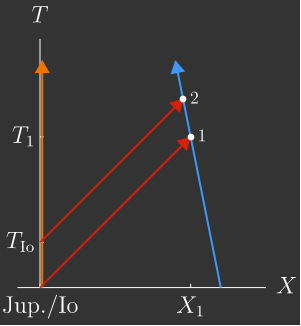If Earth is a distance of $X_1$ from Jupiter during Event 1, what are Event 1's coordinates?

Note: It is conventional in relativity to put the time coordinate of an event first; in the next chapter, we will start to see why Minkowski, an early relativist and one of Einstein's contemporaries, decided on this convention.

Propagation of Light

If Earth's position is $X_2$ at Event 2, what are its coordinates on the diagram?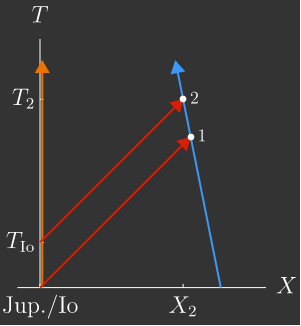Propagation of Light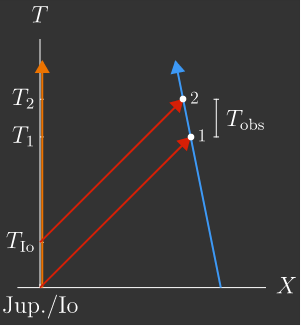Using the answers to the last two questions, the period of Io observed by someone on Earth is \begin{aligned} T_\textrm{obs} &= T_2 - T_1 \\ &= T_\textrm{Io} + \frac{X_2}{c} - \frac{X_1}{c} \\ &= T_\textrm{Io} - \frac{\Delta X}{c}\end{aligned}

where $\Delta X=X_1 - X_2$ is the change in distance from the observer to the Jupiter/Io system. Unfortunately, this distance depends on where Earth and Jupiter are in their orbits and is not easily known.

It may appear that all we've accomplished is to express the unknown $T_\text{obs}$ in terms of another unknown $\Delta X.$ However, under the assumption that Earth is moving relative toward Jupiter with constant velocity $v,$ we can express $\Delta X$ in terms of $T_\text{obs}.$

Using the definition of velocity, what is $\Delta X?$

We will use this to solve for $T_\textrm{obs}$ on the next page.

Propagation of Light

This gives us a relationship that we can solve for $T_\textrm{obs}$ in terms of $T_\textrm{Io}:$ $T_\textrm{obs} = T_\textrm{Io} - \frac{v}{c}T_\textrm{obs}.$ We find that $\boxed{ T_\textrm{obs} = \dfrac{T_\textrm{Io}}{\ 1+\dfrac{v}{c}}\ }.$ The denominator is always greater than $1$, so the period he measures is shorter than the actual period of Io, as we anticipated.

At another time during the year, when Earth is moving directly away from Jupiter and Io, Rømer makes a new measurement of Io's period. How does his new observation compare to the first?

Propagation of Light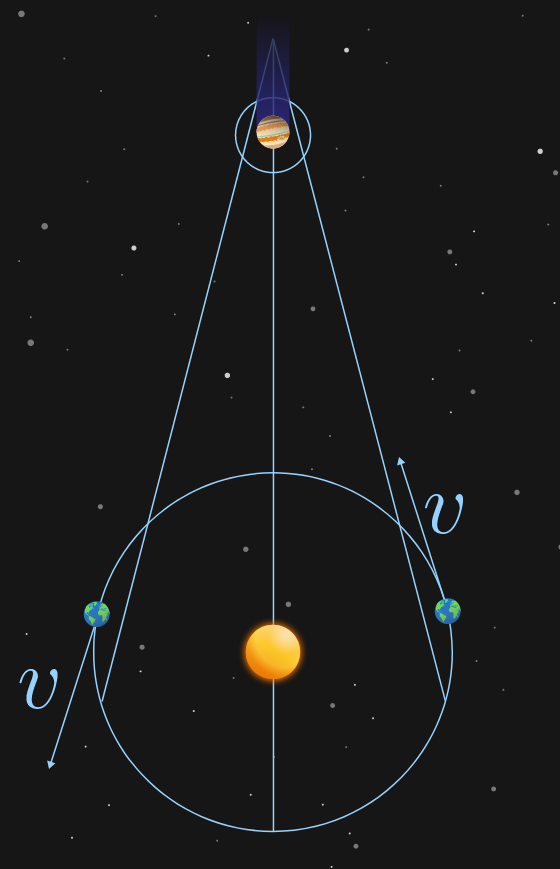Over a period of about $5$ years, Rømer made measurements of Io's period, while also tracking the relative motion between Jupiter and Earth. When Earth was receding from Jupiter, he measured longer periods; when Earth was approaching Jupiter, his measurements were shorter.

Suppose Rømer observed a difference of $\SI{30}{\second}$ between his longest and shortest observations. From this information, estimate the speed of light $c$ from your expression for $T_\text{obs}$ and use $T_\textrm{Io}=\SI{42}{\hour}.$ Enter your answer in $\si[per-mode=symbol]{\kilo\meter\per\second}$ to $4$ significant figures.

Assume the relative speed between Earth and Jupiter is roughly equal to Earth's orbital speed, $v=\SI[per-mode=symbol]{30}{\kilo\meter\per\second}.$

Propagation of Light

On the human scale, light travels astoundingly fast compared to the speeds of most material objects. This is the biggest challenge in measuring its speed. As far as most of us are concerned, light travels faster than "the blink of an eye...".

Incidentally, blinking takes about $\SI{\frac13}{\second}.$ If the circumference of Earth is about $\SI{40000}{\kilo\meter},$ how many times around the earth can light travel in the time it takes to blink?Hint: the estimate of $c$ you made in the last question is close enough to use here.

Propagation of Light

×